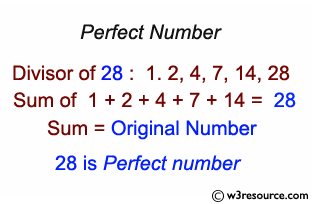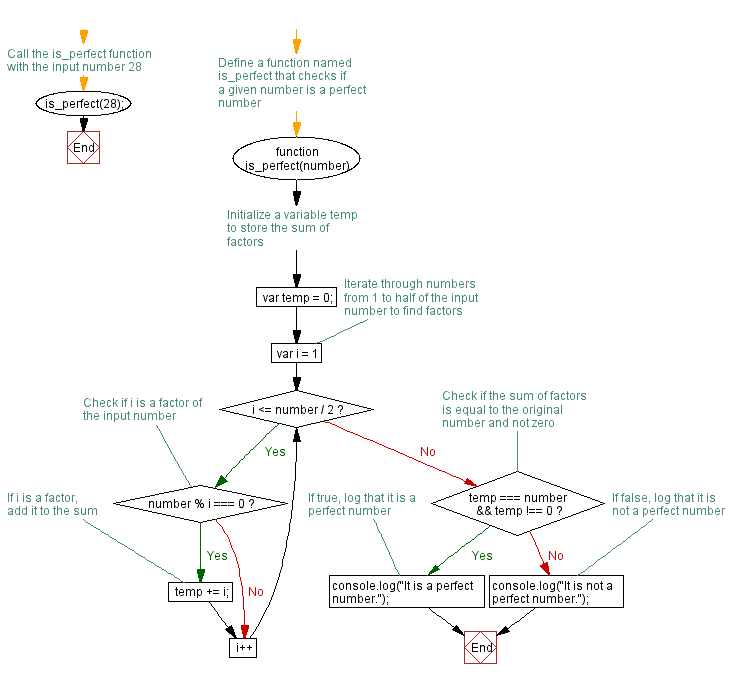# JavaScript: Find a perfect number

## JavaScript Function: Exercise-12 with Solution

Write a JavaScript function that checks whether a number is perfect.

Note : According to Wikipedia : In number theory, a perfect number is a positive integer that is equal to the sum of its proper positive divisors, that is, the sum of its positive divisors excluding the number itself (also known as its aliquot sum). Equivalently, a perfect number is a number that is half the sum of all of its positive divisors (including itself).
Example : The first perfect number is 6, because 1, 2, and 3 are its proper positive divisors, and 1 + 2 + 3 = 6. Equivalently, the number 6 is equal to half the sum of all its positive divisors: ( 1 + 2 + 3 + 6 ) / 2 = 6. The next perfect number is 28 = 1 + 2 + 4 + 7 + 14. This is followed by the perfect numbers 496 and 8128.

Pictorial Presentation:Sample Solution: -

HTML Code:

``````<!DOCTYPE html>
<html>
<meta charset=utf-8 />
<title>Find a perfect number</title>
<body>

</body>
</html>
```
```

JavaScript Code:

``````function is_perfect(number)
{
var temp = 0;
for(var i=1;i<=number/2;i++)
{
if(number%i === 0)
{
temp += i;
}
}

if(temp === number && temp !== 0)
{
console.log("It is a perfect number.");
}
else
{
console.log("It is not a perfect number.");
}
}
is_perfect(28);
```
```

Sample Output:

```It is a perfect number.
```

Flowchart:Live Demo:

See the Pen JavaScript - Find a perfect number-function-ex- 12 by w3resource (@w3resource) on CodePen.

Improve this sample solution and post your code through Disqus

What is the difficulty level of this exercise?

Test your Programming skills with w3resource's quiz.

﻿